## The Elliptic Curve Discrete Logarithm Problem

Problem 6.4 (Elliptic Curve Discrete Log Problem)   Suppose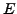is an elliptic curve over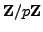and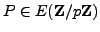. Given a multiple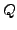of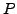, the elliptic curve discrete log problem is to find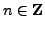such that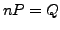.

For example, letbe the elliptic curve given by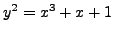over the field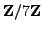. We have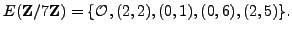If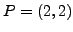and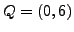, then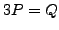, so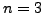is a solution to the discrete logarithm problem.

If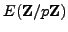has order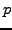or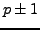or is a product of reasonably small primes, then there are some methods for attacking the discrete log problem on, which are beyond the scope of this book. It is thus important to be able to compute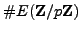efficiently, in order to verify that the elliptic curve one wishes to use for a cryptosystem doesn't have any obvious vulnerabilities. The naive algorithm to computeis to try each value of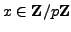and count how often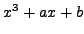is a perfect square mod, but this is of no use whenis large enough to be useful for cryptography. Fortunately, there is an algorithm due to Schoof, Elkies, and Atkin for computingefficiently (polynomial time in the number of digits of), but this algorithm is beyond the scope of this book.

In Section 3.1.1 we discussed the discrete log problem in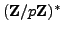. There are general attacks called index calculus attacks'' on the discrete log problem inthat are slow, but still faster than the known algorithms for solving the discrete log in a general'' group (one with no extra structure). For most elliptic curves, there is no known analogue of index calculus attacks on the discrete log problem. At present it appears that giventhe discrete log problem inis much harder than the discrete log problem in the multiplicative group. This suggests that by using an elliptic curve-based cryptosystem instead of one based onone gets equivalent security with much smaller numbers, which is one reason why building cryptosystems using elliptic curves is attractive to some cryptographers. For example, Certicom, a company that strongly supports elliptic curve cryptography, claims:

[Elliptic curve crypto] devices require less storage, less power, less memory, and less bandwidth than other systems. This allows you to implement cryptography in platforms that are constrained, such as wireless devices, handheld computers, smart cards, and thin-clients. It also provides a big win in situations where efficiency is important.''

For an up-to-date list of elliptic curve discrete log challenge problems that Certicom sponsors, see [#!certicom:challenge!#]. For example, in April 2004 a specific cryptosystem was cracked that was based on an elliptic curve over, wherehas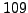bits. The first unsolved challenge problem involves an elliptic curve over, wherehas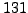bits, and the next challenge after that is one in whichhas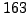bits. Certicom claims at [#!certicom:challenge!#] that the-bit challenge problem is computationally infeasible.

William 2007-06-01